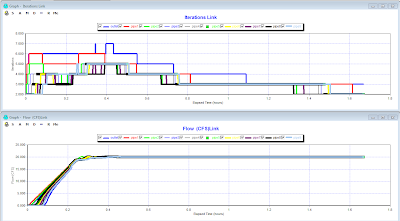### Link Iterations in the SWMM 5 Dynamic Wave Solution

Subject:   Link Iterations in the SWMM 5 Dynamic Wave Solution

Each of the links in the SWMM 5 network can use up to 8 iterations to reach convergence during a time step in the dynamic wave solution of SWMM 5.  The rules governing the number of iterations are:

1.       A minimum of 2 iterations per time step with the 1st iteration NOT using the underrelaxtion parameter of 0.5 (Figure 1)

2.       If both the downstream and upstream nodes are converged then the link drops out of the iteration process during the time step (Figure 2)

3.       The number of iterations for each link can vary over the simulation from 2 to 8 depending on how fast the flow is changing.

Figure 1.  A minimum of two and up to eight iterations per time step in the SWMM 5 dynamic wave solution.Figure 2.  The number of iterations for each link vary through out  the simulation with less iterations being used for constant flows.